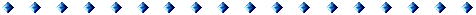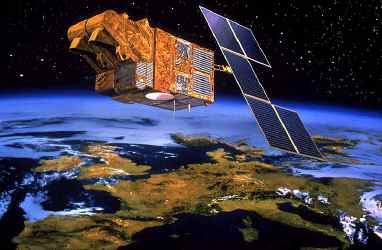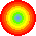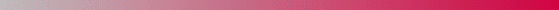Parameter estimation for satellite image deconvolution by a MCMCML methodAndré Jalobeanu - Laure Blanc-Féraud - Josiane ZerubiaClick here to begin...

 C o n t e n t s

• Introduction

•
• What is the problem?
• Reconstruction - Regularization
• Purpose of the regularization
• Purpose of the hyperparameters
• How to choose the right hyperparameters
• Choice of the estimator
• Calculus of the Likelihood
• Log-Likelihood derivatives
• Sampling the densities
• The modified Geman & Yang sampler
• The MCMCML estimation algorithm
• The ICM-DCT reconstruction algorithm
• Estimation results
• Reconstruction results

•
• Conclusion and future work
• A p p e n d i x
• Notations
• Markov random fields
• Choice of an estimation area
•André Jalobeanu - 24 Aug 1998